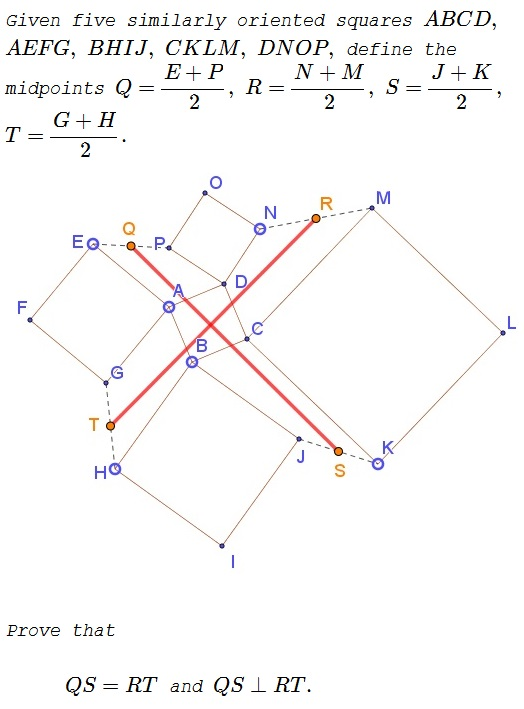# Asymmetric Propeller of Squares II

### Problem### Solution

We'll use the lower case letter corresponding to the names of of the points as associated complex numbers. We also assume, $a=1,$ $b=i,$ $c=-1,$ $d=-i.$ Then, for example, $(g-a)=i(e-a)$ so that $g=a+i(e-a).$ Similarly,

\displaystyle\begin{align} j&=b+i(h-b),\\ m&=c+i(k-c),\\ p&=d+i(n-d). \end{align}

Further,

\displaystyle \begin{align} Q&=\frac{E+P}{2}=\frac{e+p}{2}=\frac{e+d+i(n-d)}{2},\\ R&=\frac{N+M}{2}=\frac{n+m}{2}=\frac{n+c+i(k-c)}{2},\\ S&=\frac{J+K}{2}=\frac{j+k}{2}=\frac{k+b+i(h-b)}{2},\\ T&=\frac{H+G}{2}=\frac{h+g}{2}=\frac{h+a+i(e-a)}{2}. \end{align}

Further,

\displaystyle \begin{align} 2(q-s)&=[e+d+i(n-d)]-[k+b+i(h-b)]\\ &=[e+d-k-b]+i[n-d-h+b]\\ &=[e-k]+i[n-h]+2(i-1),\\ 2(r-t)&=[n+c+i(k-c)]-[h+a+i(e-a)]\\ &=[n+c-h-a]+i[k-c-e+a]\\ &=[n-h]+i[k-e]+2(i-1)\\ &=-i\{[e-k]+i[n-h]\}+2(i-1), \end{align}

because $(d-b)(1-i)=2(i-1)=(c-a)(i-1).$ It follows that $r-t=i(s-q).$

### Acknowledgment

This problem grew out of another one and was proposed by Alexandr Skutin.